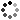# 6th Grade Math Higher Order Thinking Skills Activity - What's the Question?

On Sale
\$4.50
\$4.50
Preview

Encourage your 6th grade math students to use their higher-order thinkig skills in this activity. Students will critically think as they develop the question to the given answer. This includes 14 different 6th grade math topics. There is one topic per page. Each topic consists of 5 different “questions.” The questions are actually the answers given to five different problems, and the students have to create the questions. This will help your students think deeper about the mathematics.

The 14 topics include:
• Dividing Fractions
• Multiplying and Dividing Multi-Digit Decimals: These use factors of 10, so students are thinking about the effects of the decimal.
• GCF & LCM
• Absolute Value
• Coordinate Plane: Coordinates in each quadrant
• Simplifying Expressions: Using natural numbers and the distributive property
• Solving Equations and Inequalities: Using natural numbers
• Unit Rates: Unit rates of whole numbers
• Percents: Percents as rates and finding the whole/part given percent and whole/part
• Converting Units
• Area: Area of triangles and shapes composed of triangles and rectangles.
• Surface Area and Volume: Surface area and volume of rectangular prisms and pyramids.
• Defining Statistics: statistical questions and measures of center vs. measures of variability
• Calculating Statistics: mean, median, mode, range, and interquartile range

An answer sheet is NOT included. Due to the nature of the activity, the answers will widely vary. I have included sample answers for various questions to give the teacher an idea of what to accept. A suggestion for the teacher: do not be so strict on students writing the right “answer” for this activity. Rather this is to challenge students to think beyond basic algorithms. Focus on the thinking of the students and you can learn a great deal on what your students really understand.

These would be great to use for assessments, homework, math centers, group work, or individual work.

Please feel free to contact me with any questions!

Michelle,
Make Sense of Math